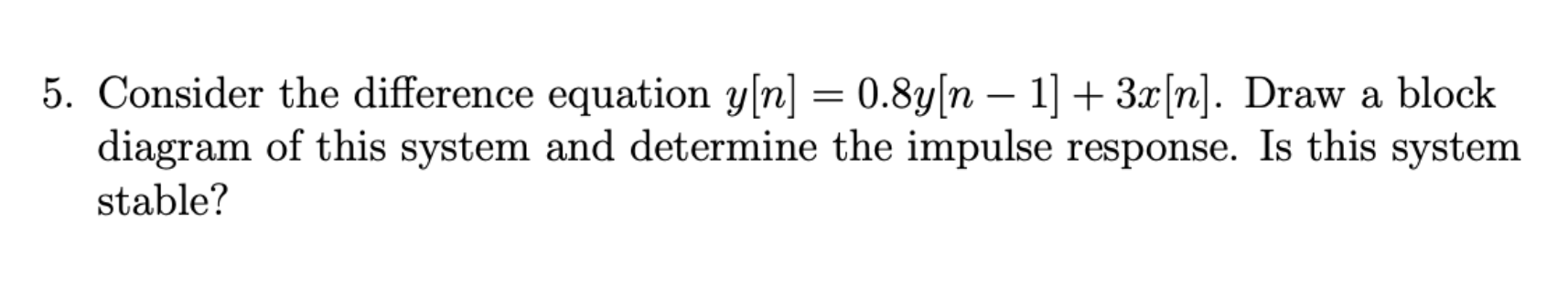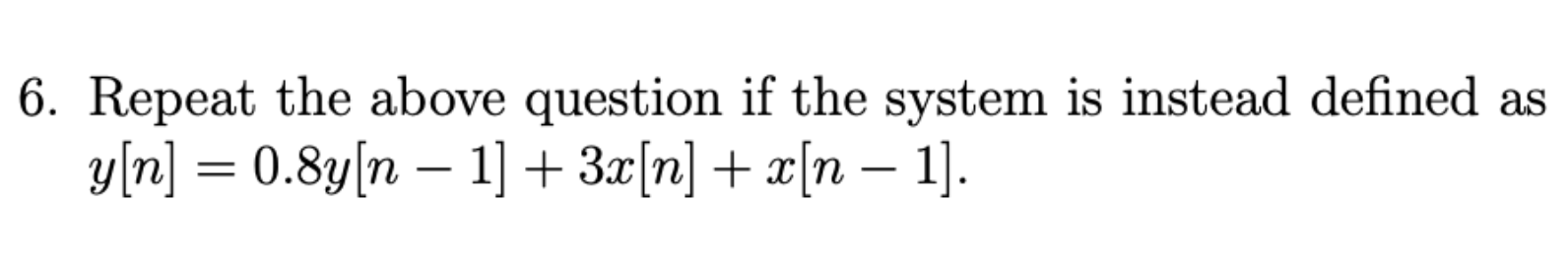Home / Expert Answers / Electrical Engineering / 5-consider-the-difference-equation-y-n-0-8y-n1-3x-n-draw-a-block-diagram-of-this-system-and-pa556

# (Solved): 5. Consider the difference equation y[n]=0.8y[n1]+3x[n]. Draw a block diagram of this system and ...5. Consider the difference equation . Draw a block diagram of this system and determine the impulse response. Is this system stable? 6. Repeat the above question if the system is instead defined as .

We have an Answer from Expert